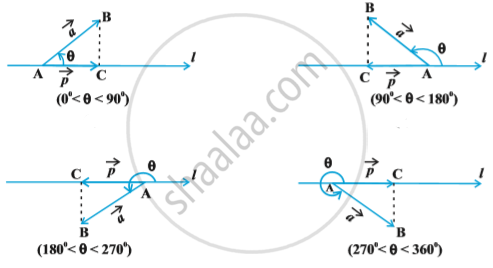# Product of Two Vectors - Projection of a Vector on a Line

## Notes

Suppose a vector vec (AB) makes an angle θ with a given directed line l (say), in the anticlockwise direction  in following fig.Then the projection of vec (AB) on l is a vector vec p (say) with magnitude |vec (AB)|  |cos θ|  , and the direction of vec p  being the same (or opposite) to that of the line l, depending upon whether cosθ is positive or negative. The vector vec p
is called the projection vector, and its magnitude |vec p| is simply called as the projection of the vector |vec (AB)| on the directed line l.
For example , in each  of the following fig. , projection vectors of vec (AB) along the line l is vector vec (AC).

Observations:
1) If hat p is the unit vector along a line l , then the projection of a vector vec a on the line l is given by vec a . hat p.

2) Projection of a vector vec a on other vector vec b, is given by
vec a . hat b, or vec a ((vec b)/(|vec b|)) , or 1/|vec b| (vec a.vec b)

3)  If  θ = 0, then the projection vector of vec(AB) will be vec (AB) itself and if θ = π, then the projection vectors of vec (AB) will be vec (BA).

4) If θ = π / 2 or θ = (3π)/2, then the projection vector of vec (AB) will be zero vector.

Remark: If α, β and γ are the direction angles of vector vec a = a_1 hat i + a_2hat j + a_3 hat k , then its direction cosines may be given as
cos alpha = (vec a . hat i)/(|vec a||hat i|) = a_1/|vec a| , cos β  = a_2/ |vec a| and cos gamma = a_3 /|vec a|

Also , note that |vec a|  cos alpha, |vec a|  cos  β  and |vec a|  cos gamma are respectively the projections of vec a along OX, OY and OZ i.e. the scalar components a_1,a_2 and a_3 of the vector vec a, are precisely the projections of vec a along x - axis , y- axis and z - axis , respectively. Further , if  vec a is a unit vector , then it may be expressed in terms of its direction cosines as
vec a = cos alpha  hat i + cos  β  hat j + cos gamma  hat k

If you would like to contribute notes or other learning material, please submit them using the button below.

### Shaalaa.com

Vector Algebra part 18 (Projection of vector on line) [00:06:24]
S
0%